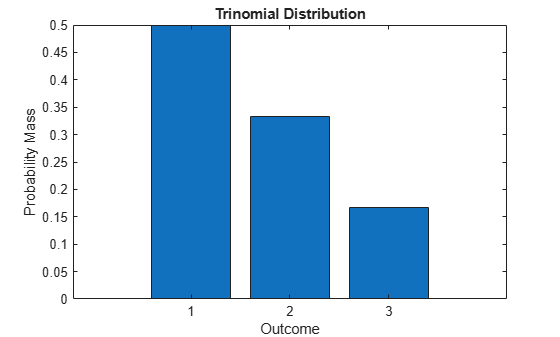Multinomial Probability Distribution Objects

This example shows how to generate random numbers, compute and plot the pdf, and compute descriptive statistics of a multinomial distribution using probability distribution objects.

Step 1. Define the distribution parameters.

Create a vector p containing the probability of each outcome. Outcome 1 has a probability of 1/2, outcome 2 has a probability of 1/3, and outcome 3 has a probability of 1/6. The number of trials n in each experiment is 5, and the number of repetitions reps of the experiment is 8.

p = [1/2 1/3 1/6];
n = 5;
reps = 8;

Step 2. Create a multinomial probability distribution object.

Create a multinomial probability distribution object using the specified value p for the Probabilities parameter.

pd = makedist('Multinomial','Probabilities',p)
pd =
MultinomialDistribution

Probabilities:
0.5000    0.3333    0.1667

Step 3. Generate one random number.

Generate one random number from the multinomial distribution, which is the outcome of a single trial.

rng('default')  % For reproducibility
r = random(pd)
r = 2

This trial resulted in outcome 2.

Step 4. Generate a matrix of random numbers.

You can also generate a matrix of random numbers from the multinomial distribution, which reports the results of multiple experiments that each contain multiple trials. Generate a matrix that contains the outcomes of an experiment with n = 5 trials and reps = 8 repetitions.

r = random(pd,reps,n)
r = 8×5

3     3     3     2     1
1     1     2     2     1
3     3     3     1     2
2     3     2     2     2
1     1     1     1     1
1     2     3     2     3
2     1     3     1     1
3     1     2     1     1

Each element in the resulting matrix is the outcome of one trial. The columns correspond to the five trials in each experiment, and the rows correspond to the eight experiments. For example, in the first experiment (corresponding to the first row), one of the five trials resulted in outcome 1, one of the five trials resulted in outcome 2, and three of the five trials resulted in outcome 3.

Step 5. Compute and plot the pdf.

Compute the pdf of the distribution.

x = 1:3;
y = pdf(pd,x);
bar(x,y)
xlabel('Outcome')
ylabel('Probability Mass')
title('Trinomial Distribution')The plot shows the probability mass for each $k$ possible outcome. For this distribution, the pdf value for any x other than 1, 2, or 3 is 0.

Step 6. Compute descriptive statistics.

Compute the mean, median, and standard deviation of the distribution.

m = mean(pd)
m = 1.6667
med = median(pd)
med = 1
s = std(pd)
s = 0.7454